# Test: Matrix Operations

## 15 Questions MCQ Test Mathematics (Maths) Class 12 | Test: Matrix Operations

Description
Attempt Test: Matrix Operations | 15 questions in 15 minutes | Mock test for JEE preparation | Free important questions MCQ to study Mathematics (Maths) Class 12 for JEE Exam | Download free PDF with solutions
QUESTION: 1

### The product of two matrics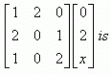Solution:

{(1*0, 2*2, 0*x) (2*0, 0*2, 1*x) (1*0, 0*2, 2*x)}
= {4, x, 2x}

QUESTION: 2

### If A, B are, respectively m × n, k × l matrices, then both AB and BA are defined if and only if​

Solution:

If A, B are, respectively m × n, k × l matrices, then both AB and BA are defined if and only if n = k and l = m. In particular, if both A and B are square matrices of the same order, then both AB and BA are defined.

QUESTION: 3

###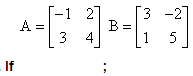and 2A + B + X = 0, then the matrix X = ……

Solution: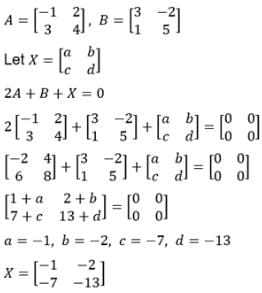QUESTION: 4

If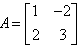then -5A = ?

Solution:
QUESTION: 5

If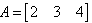and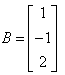, then AXB=?

Solution:

A = [2, 3, 4]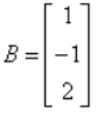Therefore AXB = {(2*1) + (3*(-1)) + (4*2)}
AXB = {2 + (-3) + 8}
AXB = 7

QUESTION: 6

If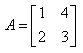and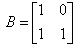, then = 2A - B?

Solution:
QUESTION: 7

If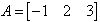and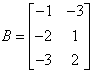, then AB = ?

Solution: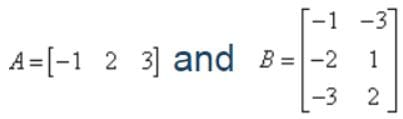A.B = [(-1(-1) + 2(-2) + 3(-3)   -1(-3) + 2(1) + 3(2)]

A.B = [1 - 4 - 9     3 + 2 + 6]

A.B = [-12   11]

QUESTION: 8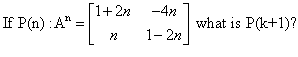Solution:

P(n) : An = {(1+2n, -4n), (n,(1 - 2n))}
= P(k + 1) = {(1+2(k+1), -4(k+1)), (k+1, (1 - 2(k+1)}
= {(1+2k+2, -4k-4) (k+1, 1-2k-2)}
= {(2k+3, -4k-4), (k+1, -2k-1)}

QUESTION: 9

Value of determinant is computed by adding multiples of one row to

Solution:

Value of Determinant remains unchanged if we add equal multiples of all the elements of row (column) to corresponding elements of another row (column) If, we have a given matrix A.

QUESTION: 10

If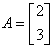and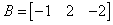, then AB = ?

Solution:

A = {(2),(3)}      B = {-1,2,-2}
AB = {(-2,4,-4) (-3,6,-6)}

QUESTION: 11

For a skew symmetric even ordered matrix A of integers, which of the following will not hold true:

Solution:

Determinant of a skew symmetric even ordered matrix A is a perfect square.

QUESTION: 12

If A is a matrix of order 1×3 and B is a matrix of order 3×4, then order of the matrix obtained on multiplying A and B is​

Solution:

In matrix 1*3 is one row and 3 columns and in 3*4 is three rows and four column hence multiplied matrix will be 1*4.

QUESTION: 13

If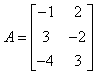and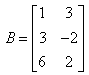, then A-2B is equal to

Solution:

A={(-1,2) (3,-2) (-4,3)}     B={(1,3) (3,-2) (6,2)}
2B = {(2,6) (6,-6) (12,4)}
A - 2B = {(-1,2) (3,-2) (-4,3)} - {(2,6) (6,-6) (12,4)}
= {(-1-2, 2-6) (3-6, -2+4) (-4-12, 3-4)}
= {(-3,-4) (-3,2) (-16, -1)}

QUESTION: 14

If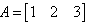and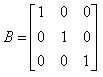then AB = ?

Solution:
QUESTION: 15

If A and B are two matrices conformable to multiplication such that their product AB = O(Zero matrix). Then which of the following can be true​

Solution:

AB = 0 does not necessarily imply that either A or B is a null matrix
- Both matrices need not be null matrices.Use Code STAYHOME200 and get INR 200 additional OFF Use Coupon Code